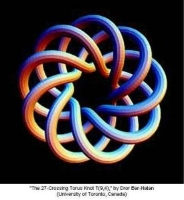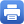Columbia Home
TopologyTopology is concerned with the intrinsic properties of shapes of spaces. One class of spaces which plays a central role in mathematics, and whose topology is extensively studied, are the n dimensional manifolds. These are spaces which locally look like Euclidean n-dimensional space.

Historically, topology has been a nexus point where algebraic geometry, differential geometry and partial differential equations meet and influence each other, influence topology, and are influenced by topology. More recently, topology and differential geometry have provided the language in which to formulate much of modern theoretical high energy physics. This interaction has brought topology, and mathematics more generally, a whole host of new questions and ideas. Because of its central place in a broad spectrum of mathematics there has always been a great deal of interaction between work in topology and work in these neighboring disciplines. That is true of the topology group at Columbia, which has enjoyed a close connection with the algebraic geometry group, the geometric PDE group, and the mathematical physics group at Columbia. In addition to close connection to the other research groups, our topology group also enjoys close collaboration with the symplectic geometers at Stony Brook and Courant, running a thrice-per-semester joint symplectic geometry seminar.

Ironically, in topology, the case of manifolds of dimensions 3 and 4, the physical dimensions in which we live, has eluded undestanding for the longest time. The case of manifolds of dimension n=1 is straightforward, and the case where n=2 was understood thoroughly in the 19th century. Moreover, intense activity in the 1960’s (including the pioneering work of Browder, Milnor, Novikov, and Smale) expresses the topology of manifolds of dimension n>4 in terms of an elaborate but purely algebraic description.

The study of manifolds of dimension n=3 and 4 is quite different from the higher-dimensional cases; and, though both cases n=3 and 4 are quite different in their overall character, both are generally referred to as low-dimensional topology.

Low-dimensional topology is currently a very active part of mathematics, benefiting greatly from its interactions with the fields of partial differential equations, differential geometry, algebraic geometry, modern physics, representation theory, number theory, and algebra.

For the case of manifolds of dimension n=3, a conjectural classification picture emerged in the 1970’s, thanks to the work of William Thurston, in terms of symmetric geometries. Specifically, Thurston conjectured that every three-manifold can be decomposed canonically into pieces, each of which can be endowed with one of eight possible geometries. Elements of this vast picture are presently unfolding thanks to the Ricci Flow equations introduced by Richard Hamilton, which have been used by Grigory Perelman to solve the century-old Poincaré conjecture, and have also shed light on Thurston’s more general geometrization conjecture. The central role of Thurston’s conjecture in three-manifold topology has helped place hyperbolic geometry, the richest of the eight geometries, into the research forefront.

The case of manifolds of dimension n=4 remains the most elusive. In view of the foundational results of Freedman, understanding manifolds up to their topological equivalence is a theory which is similar in character to the higher-dimensional manifold theory. However, the theory of differentiable four-manifolds is quite different. The subject was fundamentally transformed by the pioneering work of Simon Donaldson, who was studying moduli spaces of solutions to certain partial differential equations which came from mathematical physics. Studying algebro-topological properties of these moduli spaces, Donaldson came up with very interesting smooth invariants for four-manifolds which demonstrated the unique and elusive character of smooth four-manifold topology. In the case where the underlying manifold is Kähler, these moduli spaces also admit an interpretation in terms of stable bundles, and hence shed light on the differential topology of smooth algebraic surfaces. Since Donaldson’s work, the physicists Seiberg and Witten introduced another smooth invariant of four-manifolds. Since then, the study of four-manifolds and their invariants has undergone several further exciting developments, tying them deeply with ideas from symplectic geometry and pseudo-holomorphic curves, and hence forming further bridges with algebraic and symplectic geometry, but also connecting them more closely with knot theory and three-manifold topology.

Topology at Columbia University has enjoyed a long tradition. Illustrious professors from the past include Samuel Eilenberg, who is responsible for the foundations of algebraic topology, and Lipman Bers, whose ideas in complex variables played an influential role in Thurston’s program for three-dimensional manifolds. The senior faculty in the topology group currently are Mohammed Abouzaid, Joan Birman (Barnard emerita), Troels Jorgensen (emeritus), Mikhail Khovanov, Dusa McDuff (Barnard), John Morgan (emeritus), and Walter Neumann (Barnard emeritus). There are also a number of junior faculty, post-doctoral researchers and frequent visitors. The closest connections with research interests of other mathematicians not strictly in the topology group include David Bayer, Robert Friedman, Brian Greene, Richard Hamilton, Melissa Liu, and Michael Thaddeus. The topology group has a number of informal student seminars, a regular Topology seminar, and also a gauge theory seminar which meets on Fridays.Print this page